# RS Aggarwal Class 6 Solutions Chapter 8 Algebraic Expressions Ex 8C

## RS Aggarwal Class 6 Solutions Chapter 8 Algebraic Expressions Ex 8C

These Solutions are part of RS Aggarwal Solutions Class 6. Here we have given RS Aggarwal Solutions Class 6 Chapter 8 Algebraic Expressions Ex 8C.

Other Exercises

Question 1.
Solution:
(i) The required sum
= 3x + 7x
= (3 + 7) x
= 10x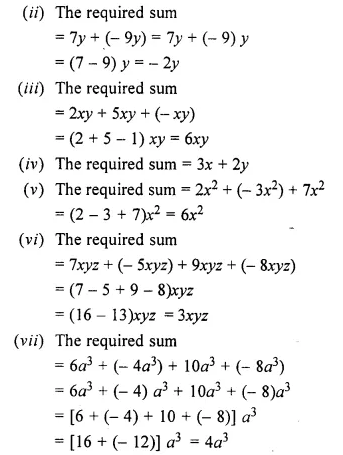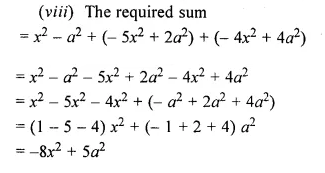Question 2.
Solution:
we get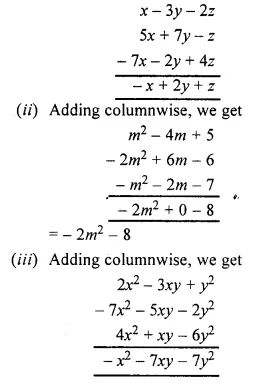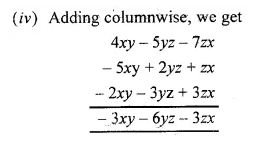Question 3.
Solution:
(i) Arranging the like terms columnwise and adding, we get :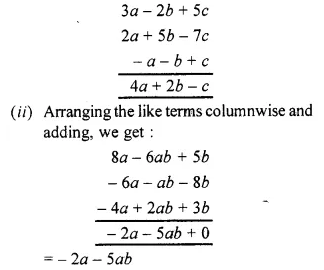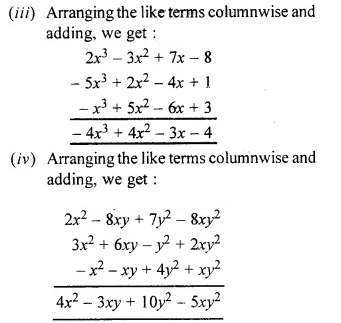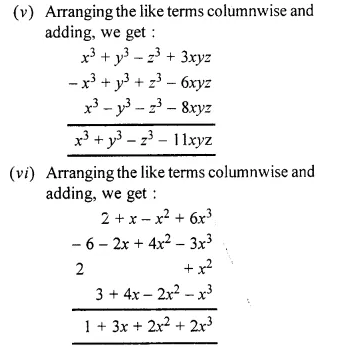Question 4.
Solution:
(i) We have :
2x – 5x = (2 – 5)x = – 3x
(ii) We have :
6x – y – (- xy) = 6xy + xy = 7xy
(iii) We have : 5b – 3a
(iv) We have : 9y – ( – 7x) = 9y + 7x
(v) We have : – 7x2 – 10x2 = ( – 7 – 10)x2
= – 17x2
(vi) We have : b2 – a2 – (a2 – b2)
= b2 – a2 – a2 + b2
= b2 + b2 – a2 – a2
= (1 + 1) b2 + ( – 1 – 1) a2
= 2b2 – 2a2

Question 5.
Solution:
(i) Arranging the like terms columnwise, we get :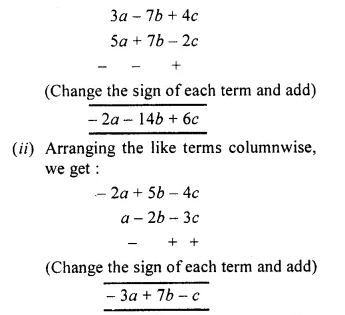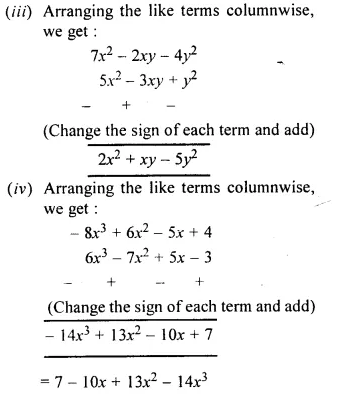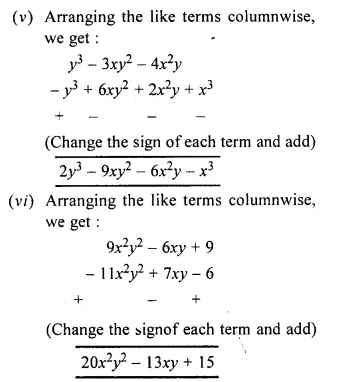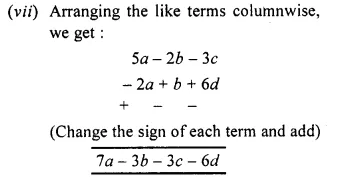Question 6.
Solution:
(i) Rearranging and collecting the like terms, we get :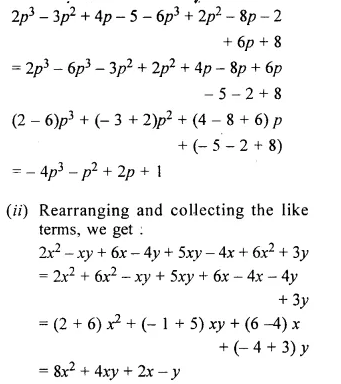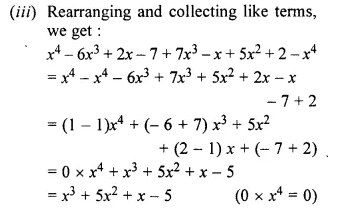Question 7.
Solution:
We have: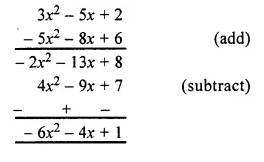Question 8.
Solution:
We have :
A = 7x2 + 5xy – 9y2
B = – 4x2 + xy + 5y2
C = 4y2 – 3x2 – 6xy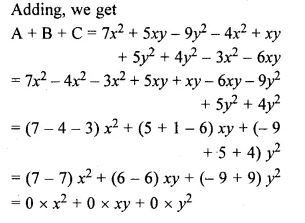= 0+0+0 = 0
Hence the result

Question 9.
Solution:
Required expression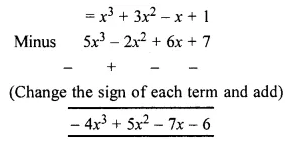Question 10.
Solution:
Substituting the values of P, Q, R and S, we have :
P + Q + R + S = (a2 – b2 + 2ab)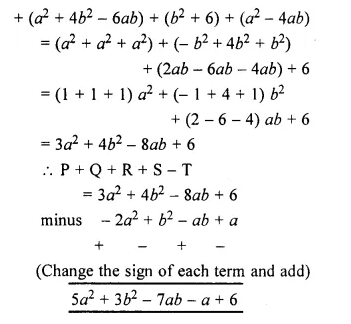Question 11.
Solution:
Required expression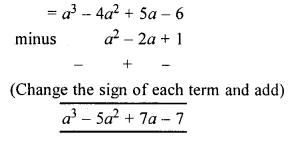Question 12.
Solution:
Required expression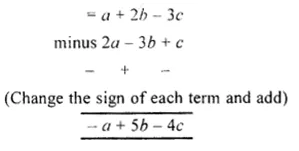Question 13.
Solution:
Required expression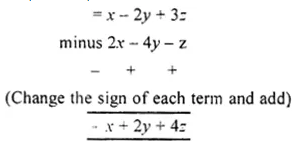Question 14.
Solution:
Required expression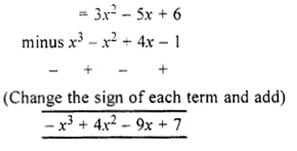Question 15.
Solution:
Sum of 5x – 4y + 6z and – 8x + y – 2z
= 5x – 4y + 6z – 8x + y – 2z
= 5x – 8x – 4y + y + 6z – 2z
= – 3x – 3y + 4z
Sum of 12x – y + 3z and – 3x + 5y – 8z
= 12x – y + 3z – 3x + 5y – 8z
= 12x – 3x – y + 5y + 3z – 8z
= 9x + 4y – 5z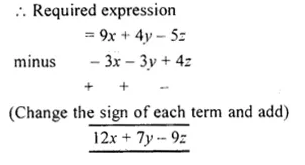Question 16.
Solution:
Required expression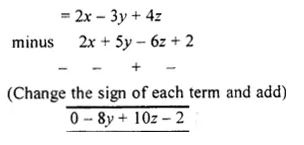Question 17.
Solution:
Required expression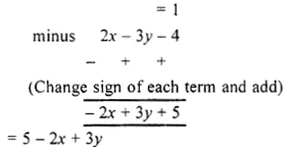Hope given RS Aggarwal Solutions Class 6 Chapter 8 Algebraic Expressions Ex 8C are helpful to complete your math homework.

If you have any doubts, please comment below. Learn Insta try to provide online math tutoring for you.